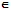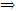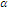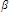# Explain vector space in algebra

Publish On: 2019-03-17

Total Post: 559

# Mjay Jollay

Total Post: 0

## ANS: Explain vector space in algebra

Let < F, +, . > be a field, the elements of F are known as scalars. Let V be a non-empty set whose elements are denoted by a, b, c, etc. and let there be two compositions on V known as internal composition {denoted by +) and scalar multiplication (external composition denoted by ‘∴’) Then the set V together with these two compositions is said to form a vector space over the field F, to be denoted by V(F), if the following axioms are satisfied:

V1: Closure property: ∀ a, bVa + bV.

V2: Associative law: ∀ a, b, cV(a + b) + c = a (b + c).

V3: Existence of identity: There exists an element 0V such that

a + 0 = a = 0 + a                ∀ aV

then, 0 is called the identity element.

V4: Existence of inverse: ∀ aV, there exists bV such that

a + b = b + a = 0.

then b is known as the inverse of a and vice versa.

V5: Scalar multiplication is associative, i.e.(a) = ()a               ∀,F andV.

V6: Commutative law: a + b = b + a

V7: Scalar multiplication is distributive over addition in F.(a + b) =a +b,            ∀ a, bV and ∀F.

V8: Distributivity of scalar multiplication over addition in F.

(+)a =a +a                   ∀,F and ∀ aV

V9: Property of unity Let 1F be the unity of F, then

1.a = a = a.1;          ∀ aV.

The elements of F are known as scalars and the elements of V are called vectors.